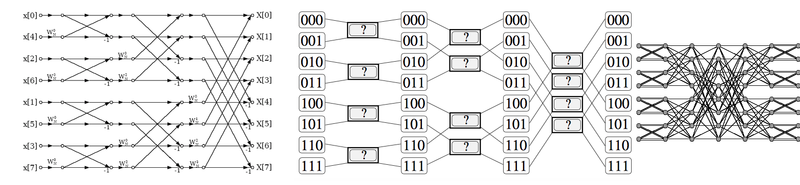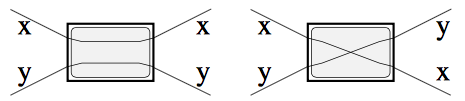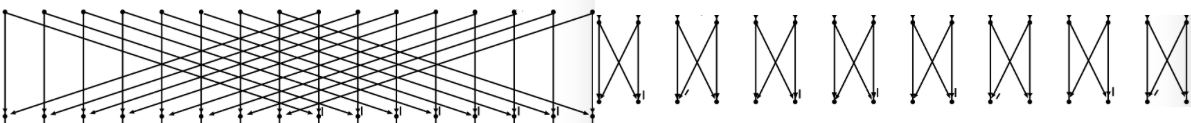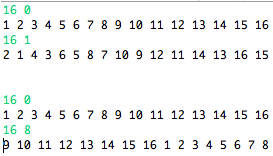Sketchy Polytopes

## 2-butterfly derangement

The hallmark of the Cooley-Tukey algorithm for
Fast Fourier Transform is the butterfly network, which helps reduce $O(n^2)$ computations to $O(n log n)$. Butterflies are very special graphs entangled in routing [Arora], switching [Chung], shuffling [Yang], and mixing [Czumaj].The butterfly with 2 inputs and outputs (aka radix-2 butterfly, or the bipartite graph $K(2, 2)$ is a building block for larger networks [Chung]. It can implement two distinct operations: pass-through and swap.Problem 1: Can an array of size $N$ be permuted using only swapping 2-butterflies in a single stage? Single stage implies that every element is swapped exactly once.

Clearly, it can be iff $N$ is even (otherwise the mapping is not bijective). Each permutation is also a maximal matching on the $K(N/2, N/2)$ spanning $N$ elements.

Problem 2: Can an array be deranged using only swapping 2-butterflies in a single stage?

There are always at least two derangements for any even-sized array when $N > 2$ (only one if $N = 2$). One can be obtained by swapping $N/2$ non-overlapping pairs. Another, by swapping the two halves of the array. Here’re two for $N = 16$:Problem 3: How many such derangements are possible?

Let $N = m * 2^k$, where $m >= 1$ is some odd integer, and $k > 0$. There are at most $log(k)$ - or, $log(N)$, if $m = 1$ - distinct derangements.

Problem 4: Is there a permutation of the first $N$ natural numbers, $[1..N]$, such that if a number $x$ is mapped to a number $y$ in the permutation, then $| x - y | == K$ for all x and y, and for a given $0 < K < N$? $|.|$ denotes the absolute value. In other words, is there a bijective function f mapping $[1..N]$ to $[1..N]$ such that $|x - f(x)| == K$?Since K > 0, this permutation must be a derangement. The previous two examples for N = 16 illustrate K = 1 and K = 8. They represent a derangement that swaps N / K groups of K neighbors with each other. If K leaves an even factor to N, and K <= N/2, then such a derangement always exists. Here’s a short program to print them out:

[code language=”java”] public static String permute(int n, int k) { StringBuilder result = new StringBuilder(2 * n); if (n % 2 == 0 && k > 0 && k <= (n / 2)) { // any k that leaves an even factor is fine // e.g. k = 4 for n = 12 is not valid but k = 2 is if ((n % k == 0) && ((n / k) % 2 == 0)) { // groups of k are swapped with neighbors int k2 = k * 2; for (int g = 0; g < n / k2; ++g) { int f = k2 * g; for (int i = k + 1; i <= k2; ++i) { result.append(f + i).append(“ “); } for (int i = 1; i <= k; ++i) { result.append(f + i).append(“ “); } } return result.toString(); } } return “No permutation exists”; } [/code]

• Arora S. Arora, F. T. Leighton, B. M. Maggs. Online algorithms for path selection in a nonblocking network.
• Chung F. R. K. Chung. An algebraic approach to switching networks.
• Yang Q. Yang, J. Ellis, K. Mamakani, F. Ruskey. In-place permuting and perfect shuffling using involutions.
• Czumaj A. Czumaj. Random permutations using __switching networks.